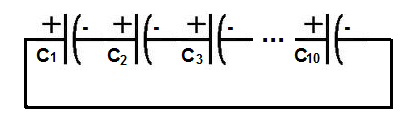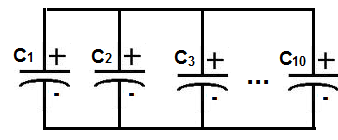﻿ Series and Parallel Capacitor Calculator ﻿# Series and Parallel Capacitor Calculator

### Series Capacitor CalculatorThis Series Capacitor calculator calculates the total series capacitance of a circuit. This calculator allows up to 10 different capacitor values. If you want to compute the total capacitance of less than 10 capacitors, just insert the values of the capacitors you have and leave the rest of tbe fields blank. For example, if you want to compute the series capacitance of 3 Capacitors, just insert values into the first 3 boxes and leave the rest blank. The calculator will give you the total series capacitance for those 3 Capacitors. If you want to compute the series capacitance of more than 10 capacitors, then just start with the first 10 capacitors and then calculate the equivalent series capacitance. Once you have this result, place it into one of the boxes and insert into the other boxes the remaining capacitors you have in series. The result will be the same as if you calculated all the capacitors at once.

For this calculator, the user has the option of choosing whether the capacitance is in farads(F), millifarads (mF), microfarads (μF), nanofarads (nF), or picofarads (pF). 1 millifarad equals 0.001 farads (10-3) (F). 1 microfarad equals 0.000001F (10-6). 1 nanofarad equals 0.000000001 (10-9). 1 picofarad equals 0.000000000001F (10-12). The user can enter the capacitance in any given unit.

This series capacitor calculator calculates the total capacitance, based on the formula above. The unit of the result which it gives is in unit farads (F).

### Parallel Capacitor CalculatorThis parallel capacitor calculator calculates the total parallel capacitance of a circuit. This calculator allows up to 10 different capacitor values. If you want to compute the total capacitance of less than 10 capacitors, just insert values of the capacitors you have and leave the rest of the fields blank. For example, if you want to compute the parallel capacitance of 3 capacitors, just insert values into the first 3 boxes and leave the rest blank. The calculator will give you the total parallel capacitance for those 3 capacitors. If you want to compute the parallel capacitance of more than 10 capacitors, then just start with the first 10 capacitors and then calculate the equivalent parallel capacitance. Once you have this result, place it into one of the boxes and insert into the other boxes the remaining capacitors you have in parallel. The result will be the same as if you calculated all the capacitors at once.

Just like the other calculator, the user has the option of entering the capacitance in different unit values, including farads(F), millifarads (mF), microfarads (μF), nanofarads (nF), or picofarads (pF).

This parallel capacitor calculator calculates the total capacitance, based on the formula above. The unit of the result which it gives is unit farads (F).

In parallel, the values of capacitors simply add up. So, for example, if there are 3 capacitors in parallel and each are 1nF each, the total equivalent capacitance value is 3nF.

Related Resources

﻿